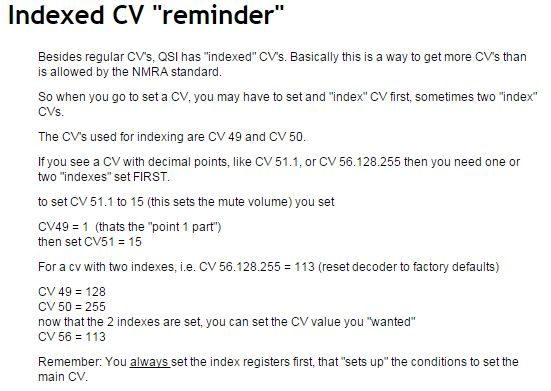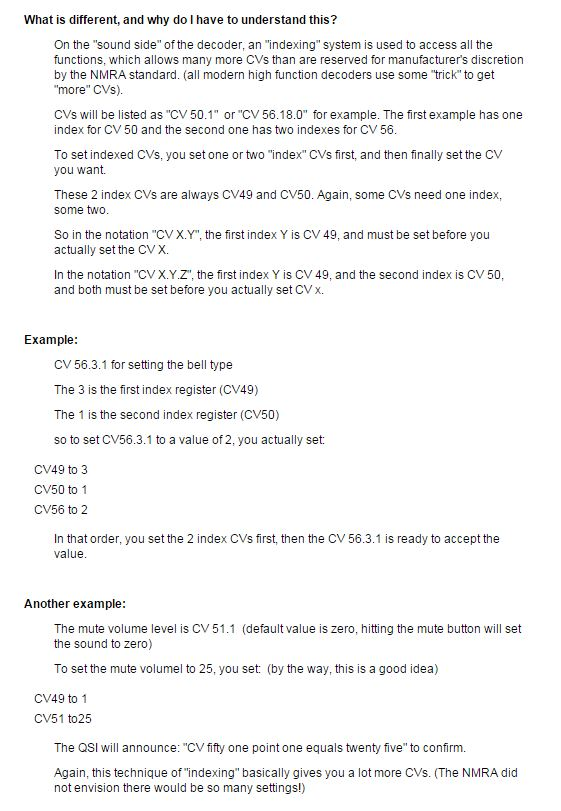Follow

# Indexed CVs - Speaking QSI

QSI uses indexed CV which requires multiple CV coordinated to access certain commands. Changing an Indexed CV is NOT complicated!  It only takes a bit longer.  Think of an Indexed CV as a combination lock.

The Indexed CV ( lock combination ) is two numbers, or sometimes three.

We are still using CVs, just using more of them.

Example:  To reset a QSI decoder to factory settings you set

Indexed CV 56.128.255 to a value of 113.  Do not think of this number as using decimal points. Those are just dots to separate the numbers.

Note that the Indexed CV has three parts, so that means three CVs need to be changed.

The first number is 56. This is the actual Indexed CV we are trying to change. We use this part of the CV last.  The value is 113.

The second number is the Primary Index. This is the CV we set FIRST.  The Primary Index CV is 49. In our example above you are setting CV 49 to a value of 128.

The third number is the Secondary Index. This is the CV we set second. The Secondary Index CV is 50. In our example above you are setting CV 50 to a value of 255.

Getting back to our combination lock analogy:

Step one - Set CV49 to 128

Step two - Set CV50 to 255

Step three -  Set CV56 to 113

http://www.elmassian.com/dcc/specific-manufacturers/qsi-equipment/qsi-cheat-sheet